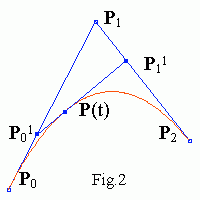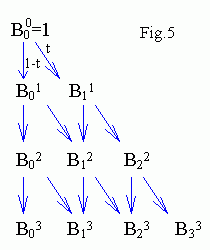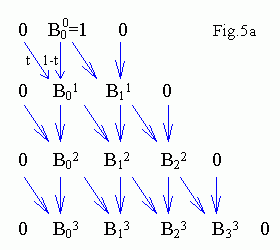# Bezier spline curves

Linear Bezier spline

Linear Bezier spline is obtained by linear interpolation between two control points P0 , P1
P(t) = (1-t)P0 + tP1 ,     0 ≤ t ≤ 1An interactive Java applet. Drag the mouse to move the nearest control point (a small blue square). In the right applet window you see basis polynomials of the linear Bezier spline. The red line is (1-t) and the green one is t.
(Sorry if your browser doesn't support Java.)

Quadratic Bezier spline is obtained by deCasteljau algorithm as a linear interpolation between linear interpolation between control points P0 , P1 , P2
P01 = (1-t)P0 + tP1 ,     P11 = (1-t)P1 + tP2 ,
P(t) = (1-t)P01 + tP11 = (1-t)[(1-t)P0 + tP1] + t[(1-t)P1 + tP2] = (1-t)2P0 + 2(1-t)tP1 + t2P2 ,

P(t) = ∑i=0,2 Bi2(t) Pi
where Bin(t) are Bernstein polynomials (see below).You see basis polynomials B02(t) , B12(t) , B22(t) in the right applet window (in red, green, blue, magenta... order).
By construction Bezier spline goes through its terminal control points, i.e.
P(0) = P0 ,     P(1) = P2 .
Note that P(t) subdivides the curve in two quadratic splines (see Fig.2). The new curves match the original in position, although they differ in parameterization. Points P0 , P01 , P(t) and P(t) , P11 , P1 are control points of the new small splines (because e.g. two quadratic splines P(t) and P'(t') determined by control points P0 , P1 , P2 and P0 , P01 , P(t) both go through two points P0 , P(t) and have the same derivative d/dt at P0 ).

Cubic Bezier spline

In a similar way one can use deCasteljau algorithm to interpolate a curve between (n+1) control points P0 , P1 ... Pn . E.g. for n = 3 we get cubic Bezier splinePoints P0 , P01 , P02 , P(t) and P(t) , P12 , P21 , P3 are control points of new small splines again. The control points of the two new curves appear along the sides of the systolic array (see Fig.4 below).

How to plot Bezier spline

DeCasteljau iterations
Pij = (1-t)Pij-1 + tPi+1j-1,     j = 1, n     i = 0, n-j
for n = 3 are shown on the scheme in Fig.4This algorithm is programmed as (see Bezier.java too)

```   for (j = N-1; j > 0; j--)
for (i = 0; i < j; i++){
Px[i] = (1-t)*Px[i] + t*Px[i+1];  Py[i] = (1-t)*Py[i] + t*Py[i+1];
}
```
where N = n+1 is number of control points.
To obtain basis polynomials recurrence relations can be used
B00(t) = 1,
Bin(t) = (1-t)Bin-1(t) + tBi-1n-1(t),

where Brs = 0 when r < 0 or r > s.
This algorithm is shown on the scheme in Fig.5a . It is programmed as
```   B = 1;
for (j = 1; j < N; j++)
for (i = j+1; i > 0; i--)
B[i] = (1-t)*B[i] + t*B[i-1];
```
here B = 0 is added for convenience, therefore i -> i+1. Basis functions can be obtained too without "zeros" (see Fig.5)
```   B = 1;
for (j = 0; j < n; j++)
for (i = j; i => 0; i--){
B[i+1] += t*B[i];
B[i] += (1-t)*B[i];
}
```
Bezier spline of degree n = 7

The general expression for Bezier spline of degree n (order n+1) is
P(t) = ∑i=0,n Bin(t) Pi ,     (*)
Bin(t) = Cni ti(1-t)n-i ,     Cni = n! / i!(n-i)!

where Bin(t) are Bernstein polynomials.
From deCasteljau algorithm and Fig.4' it follows, that the control point P1 appears in P(t) with coefficient
t(1-t)2 + (1-t)t(1-t) + (1-t)2t = 3t(1-t)2.
We see from (*) that this coefficient is B13 too. As since we get the same B13 = 3t(1-t)2 value from Fig.5', therefore deCasteljau iterations are equivalent to formula (*).Further you can read more formal proof of the deCasteljau algorithm in More Bezier splines Math or go directly to Interpolating Lagrange curve.
Contents   Next: More Bezier splines Math
updated 7 August 2001